16-reel slot machines

2. Case B – different numbers of stops and symbol distributions on the reels

2.7  A specific symbol at least three times on a payline

The probability of this event is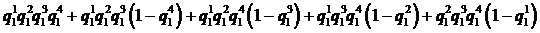, where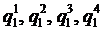are the basic probabilities of that symbol occurring on the reels respectively.

2.9  A specific symbol four times or another specific symbol at least three times on a payline

The probability of this event is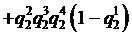, where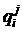are the basic probabilities of the two symbols occurring on the reels respectively.

2.10  A specific symbol four times or any combination of that symbol with another specific symbol on a payline

The probability of this event is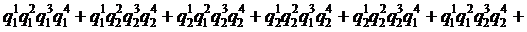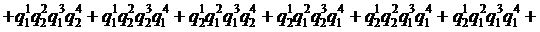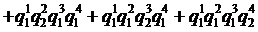, whereare the basic probabilities of the two symbols occurring on the reels respectively.